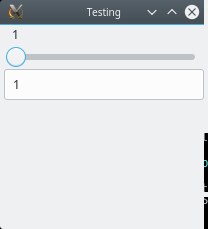A first example: GUI controls

# A first example: GUI controls

Let's create a `slider` object:

``````julia> using Gtk.ShortNames, GtkReactive

julia> sl = slider(1:11)
Gtk.GtkScaleLeaf with Signal{Int64}(6, nactions=1)

julia> typeof(sl)
GtkReactive.Slider{Int64}``````

A `GtkReactive.Slider` holds two important objects: a `Signal` (encoding the "state" of the widget) and a `GtkWidget` (which controls the on-screen display). We can extract both of these components:

``````julia> signal(sl)
Signal{Int64}(6, nactions=1)

julia> typeof(widget(sl))
Gtk.GtkScaleLeaf``````

(If you omitted the `typeof`, you'd instead see a long display that encodes the settings of the `GtkScaleLeaf` widget.)

At present, this slider is not affiliated with any window. Let's create one and add the slider to the window. We'll put it inside a `Box` so that we can later add more things to this GUI (this illustrates usage of some of Gtk's layout tools:

``````julia> win = Window("Testing") |> (bx = Box(:v));  # a window containing a vertical Box for layout

julia> push!(bx, sl);    # put the slider in the box, shorthand for push!(bx, widget(sl))

julia> showall(win);``````

Because of the `showall`, you should now see a window with your slider in it:The value should be 6, set to the median of the range `1:11` that we used to create `sl`. Now drag the slider all the way to the right, and then see what happened to `sl`:

``````julia> sl
Gtk.GtkScaleLeaf with Signal{Int64}(11, nactions=1)``````

You can see that dragging the slider caused the value of the signal to update. Let's do the converse, and set the value of the slider programmatically:

``julia> push!(sl, 1)  # shorthand for push!(signal(sl), 1)``

Now if you check the window, you'll see that the slider is at 1.

Realistic GUIs may have many different widgets. Let's add a second way to adjust the value of that signal, by allowing the user to type a value into a textbox:

``````julia> tb = textbox(Int; signal=signal(sl))
Gtk.GtkEntryLeaf with Signal{Int64}(1, nactions=2)

julia> push!(bx, tb);

julia> showall(win);``````Here we created the textbox in a way that shared the signal of `sl` with the textbox; consequently, the textbox updates when you move the slider, and the slider moves when you enter a new value into the textbox. `push!`ing a value to `signal(sl)` updates both.

Another example of connecting a widget with a signal is using a `button` to allow updates to propagate to a second `Signal`:

``````a = button("a")
x = Signal(1)
y_temp = map(sin, x)    # see the Reactive.jl documentation for info about using `map`
y = map(_ -> value(y_temp), a)``````

Subsequent `push!`es into `x` will update `y_temp`, but not `y`. Only after the user presses on the `a` button does `y` get updated with the last value of `y_temp`.